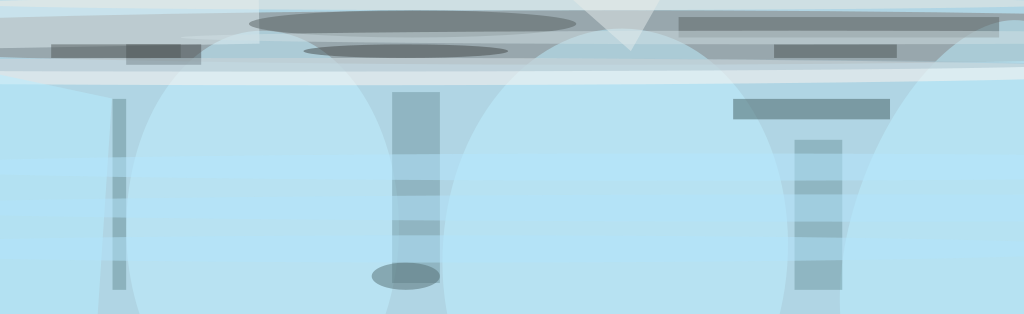A method of computing the concentrations of chemical species in chemically reacting flows includes, among other things, utilization of a prior chemical-kinetic method based on a concept of intrinsic low-dimensional manifolds (ILDM) in composition space. The present method is particularly suitable for predicting concentrations of nitrogen oxides (NOx) generated in the combustion of hydrocarbon fuels in flows simulated by techniques of computational fluid dynamics (CFD).

The composition of a chemical system can be regarded as a point in composition space, which is a multidimensional space in which each dimension represents the concentration of one of the chemical species present. The chemical-kinetic rate equations of the system can be regarded as representing how the composition point moves in the space. In a typical case, the composition changes much more rapidly in some dimensions than in others, so that the composition point can be regarded as (1) first moving rapidly toward an attracting manifold of reduced dimensionality (for example, a surface in space of three or more dimensions), then (2) moving along the manifold toward an equilibrium point.Concentrations of NOx as predicted by the present method approximated those measured in experiments in five test cases of combustion of hydrogen jets injected radially into a can downstream of an air inlet. The differences between predicted and experimental values for cases 2 through 5 should be analyzed with caution because the CFD computations for those cases were not carried out to full convergence.

In the ILDM method, one identifies such a manifold, assumes that the composition always lies on the manifold, and uses the reduced dimensionality of the manifold to reduce the amount of computation needed to solve the corresponding equations of chemical kinetics. One still accounts for all of the chemical species of a complex system, but takes advantage of the simplification to parameterize the system by use of relatively few variables. In principle, the manifold can be identified once the number of its dimensions is known or assumed.

In the present method, the ILDM method is implemented by a computer code that automatically identifies a manifold on which a simplified mathematical model of a chemical system is parameterized by two variables: (1) a mixture fraction that is a function of mass fractions of species present in the system and (2) a progress variable, which can be either another function of species mass fractions, a temperature, or a Gibbs function. The only restriction on the choice of the progress variable is that this variable must be a single-valued function in the given system. The resulting species concentrations, rates, and properties are stored in look-up tables. These tables can then be used as the chemistry model in CFD calculations. By using look-up tables, the calculation of properties and reaction rates is replaced by interpolation, resulting in significant savings in central processing unit (CPU) time.

The method can also be used to postprocess results of CFD simulations of reacting flows and, in particular, of flows in combustors burning hydrocarbon fuels in air. Typically, a CFD code yields the flow velocities, temperatures, and concentrations of the major chemical species (fuel, O2, N2, H2O, CO, and CO2). For each node on a computational grid, the mixture fraction and progress variable can be calculated from the CFD output data. Then with the help of interpolation routines that can be called from the CFD code, the mixture fraction and progress variable can be used to obtain concentrations of such minor species as NOx (see table). This computation amounts to a mapping procedure that can be accomplished rapidly; computations for a million nodes take only a few minutes.

This work was done by A. T. Norris of the Institute for Computational Mechanics in Propulsion for John H. Glenn Research Center. Inquiries concerning rights for the commercial use of this invention should be addressed to

###### John H. Glenn Research Center, Commercial Technology Office, Attn: Steve Fedor, Mail Stop 4-8, 21000 Brookpark Road, Cleveland, Ohio 44135

Refer to LEW-16659.##### NASA Tech Briefs Magazine

This article first appeared in the May, 1999 issue of NASA Tech Briefs Magazine.

Read more articles from the archives here.